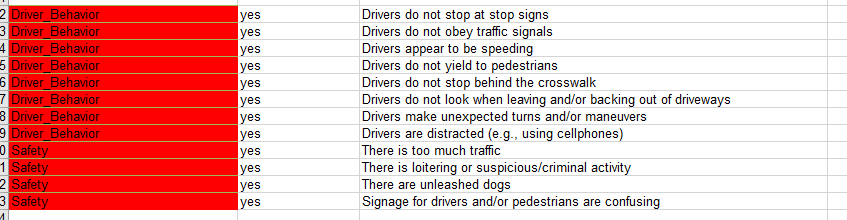# Can you calculate the sum of a multiple choice answer in Survey 123?

3587
9
06-09-2017 06:01 AMOccasional Contributor

I have a set of select_multiple questions (which have 4 answers to choose from including "Yes" and "No") on a form in Survey 123. I would like to be able to calculate the total number of yes answers automatically at the end of the survey in it's own box. Is this possible?

Tags (1)
1 Solution

Accepted SolutionsbyEsri Esteemed Contributor

Hi Michael,

Yes this is possible.  Essentially, the calculation for the count question would be a sequence of:

if(selected(\${question}, 'yes'), 1, 0)

added together - so if you had 3 questions, it looks like:

if(selected(\${question1}, 'yes'), 1, 0) + if(selected(\${question2}, 'yes'), 1, 0) + if(selected(\${question3}, 'yes'), 1, 0)

9 RepliesbyEsri Esteemed Contributor

Hi Michael,

Yes this is possible.  Essentially, the calculation for the count question would be a sequence of:

if(selected(\${question}, 'yes'), 1, 0)

added together - so if you had 3 questions, it looks like:

if(selected(\${question1}, 'yes'), 1, 0) + if(selected(\${question2}, 'yes'), 1, 0) + if(selected(\${question3}, 'yes'), 1, 0)Occasional Contributor

Thank you James, that's what I needed. If I also wanted to calculate the "no" values as well with the 'yes', would I need to do separate sequences of    if(selected(\${question}, 'no'). 1, 0) or can I combine 2 answers into 1 equation?byEsri Esteemed Contributor

Hi Michael,

Yes you would need separate sequences of no evaluations - the selected() function can only look at one answer possibility at a time.New Contributor II

Hi James,

I have the same situation where I need to calculate the sum of a couple multiple selection questions. But for each of my question, when there are multiple 'yes', it only gets counted one time.I used --- if(selected(\${Driver_Behavior},'yes'),1,0)+if(selected(\${Safety},'yes'),1,0)

See above, I want all of the checked answer to be counted. How do I achieve that? It seems like if(selected(\${question}, 'yes'), 1, 0) only checks if at least one item is checked.

BestbyEsri Notable Contributor

Hi. I think your situation is slightly different. You cannot have repeated names in a list. That is, your can't have yes be the name for all choices in your list.  That is not syntactically correct in XLSForms.

I believe in your case you may consider giving each choice a different arbitrary value, such as 1,2,3,4 etc  and then simply do a count-selected(\${yourSelect_multipleQuestionHere} against your select_multiple question.New Contributor II

Hi Ismael,

Thank you very much for pointing that out! It's working now!!!!

Cheers,by Anonymous User
Not applicable

Hello James! James Tedrick

Thank you for clarifying the method above. My question is, if there are alot of 20 questions which will have the same limited multiple choices (eg. 4 choices; A, B, C, D). Is there any other possible way to 'filter' and sum the different choices accordingly without having to type such as you've suggested:

if(selected(\${question1}, 'yes'), 1, 0) + if(selected(\${question2}, 'yes'), 1, 0) + if(selected(\${question3}, 'yes'), 1, 0)

We're doing very detailed reef substrate checking.  We have 4 segments (which I conveniently separated into groups in this survey). Each segments have 20 intervals which are the 20 questions I've mentioned earlier. And each question ('select_one' question) has 10 choice of answer! Just explaining this is already scaring me a little.

P/S: I don't even know where to type the function you've shared although I understand the gist of the solution.

Any sort of input would be super awesome and will really help us out.

AzaleaOccasional Contributor

Got it. I guess I have a few more sequences to write. Thanks again for your help James.

MichaelNew Contributor III

Hi,

What if I have a choice list of 500 trees, and a select one question in a repeat where I can chose a tree, then enter info like diameter breast height (dbh), and then calculate the basal area.  Then outside the repeat, I want to sum all the dbh values for each species choses, and also average the basal area calculations from inside the repeat.  I have done this on a smaller scale (24 trees), by giving all the trees a number, number(if(selected(\${trees},'White_Oak'),1,0)),  but when I want to do all of the tree species nation wide this could get cumbersome, thoughts?